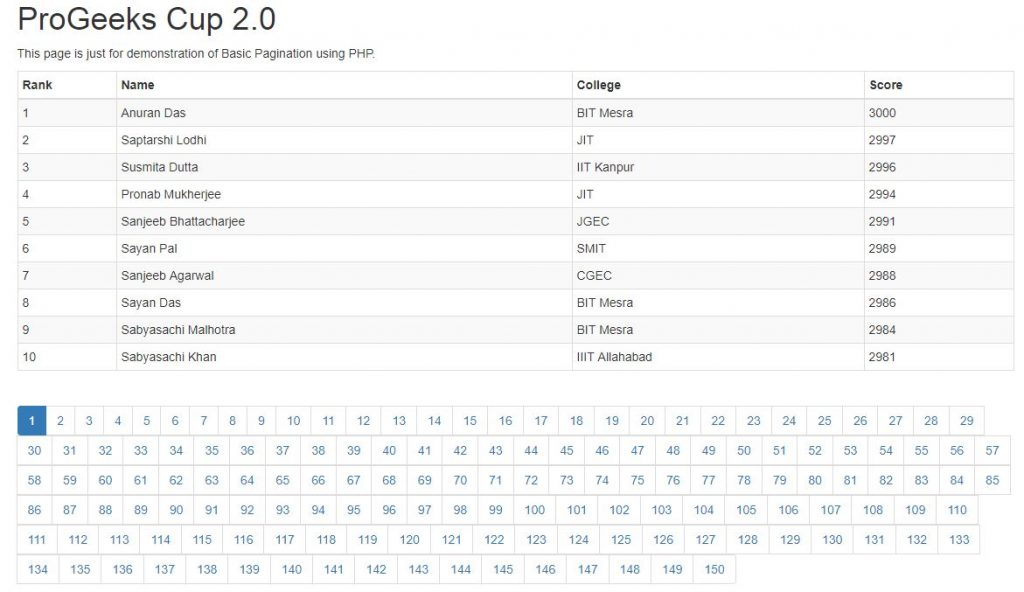Open In App

# PHP Pagination | Set 2

In the previous article on PHP Pagination, we had seen why pagination is needed and also developed a basic structure for implementing a pagination system using PHP. In continuation, let us start with our development process and first create a script to connect to our Database.

Connecting to Database

Connecting to Database is often a required task for showing data in any PHP file, thus writing the same code repeatedly is not the best approach so following the convention we will create a PHP file to be known as “connection.php”, and will write the following code.

 ``

In this article, we will be using mysql extension, in case you want to work your way with the mysqli extension please refer the following article. The above code snippet just defines the credentials such as Host, Username, Password and Database name as PHP constants and uses them to establish the connection. Now we have to fetch the data and distribute it in pages.

Data Fetch and Representation

So far, we have created a Demo Markup, we have created the Database table to hold fake data and we have also connected to the database. Now all that is left is to fetch the rows of information and show it on various pages. Now, what could be the general logic behind it. Pagination generally limits the number of entries in a page and also maintains the sequence of all the entries throughout the pages. So, if we could have a variable that would denote us the Max number of entries that can be shown on a page and given a page number we could find exactly which entries are to be shown. For example, if the current page number is 5 and the limit is 10 then we can see manually that the first page will contain 1-10, the second page will contain 11-20, and similarly, the fifth page will contain 41-50 entries.
So we could write that if the page number is denoted using \$pn and limit is denoted using \$limit then the starting index of the entry can be written as: ((\$pn-1)*\$limit)+1. As we have calculated the relation we just now need to implement the following in our “index.php” file. The following is what we should finally get.

 `````  ````    ``ProGeeks Cup 2.0``    ````    ````    ````  ````  ````  ````  ``
``    ``
``    ``
``      ``

ProGeeks Cup 2.0

``      ``

This page is just ``for` `demonstration of ``                 ``Basic Pagination using PHP.

``      ````        ``
RankNameCollegeScore
``        ````          ````          ````          ````          ````        ````        ````        ``
``        ``  ``        ``  ``          ``  ``          ````          ````          ``                                        ``        ``  ``        ``  ``        ````      ````      ``
``    ````  ````  `````

As you can see we have added three sections (highlighted parts) in the previously written code to fetch and show the data as well as links to next pages. Let us go through all these sections separately to get an in-depth understanding of the code.

Fetching from Database

 `// Import the file where we defined the connection to Database.  ``require_once` `"connection.php"``;``  ` `\$limit` `= 10;  ``// Number of entries to show in a page.``// Look for a GET variable page if not found default is 1.  ``if` `(isset(``\$_GET``[``"page"``])) { ``    ``\$pn`  `= ``\$_GET``[``"page"``]; ``} ``else` `{ ``    ``\$pn``=1; ``};  ``  ` `\$start_from` `= (``\$pn``-1) * ``\$limit``;  ``  ` `\$sql` `= ``"SELECT * FROM table1 LIMIT \$start_from, \$limit"``;  ``\$rs_result` `= mysql_query (``\$sql``); `

After we have created the connection to the Database in “connection.php” we will just import the file in with the require_once keyword. Next, we are explicitly defining the maximum number of records to be shown in a single page. Subsequently, we are checking if there is any “page” attribute in the URL i.e. we are looking for the “page” attribute in “GET” method, which if found is set to be the page number to be shown, otherwise it’s the first page or the value is 1. Following this we are calculating the index to start our fetch, it is calculated using the formulae we derived before but as SQL uses 0-indexing we are not adding the additional 1. Lastly, we are just fetching every field of the records starting from index \$start_from and limiting up to \$limit records and storing in the \$rs_result variable.

Showing the Records

 `  ``  ``
``    ``  ``    ````    ````    ``                                       ``  ``  ```

This section is fairly simple, we are just iterating over the records we fetched and fetching each row as an Associative array, we are echoing each field as table data(td tags) in each table rows(tr tags). After every iteration we are creating a table row consisting of four columns namely Rank, Name, College and Score.

 `\$sql` `= ``"SELECT COUNT(*) FROM table1"``;  ``\$rs_result` `= mysql_query(``\$sql``);  ``\$row` `= mysql_fetch_row(``\$rs_result``);  ``\$total_records` `= ``\$row``;  ``// Number of pages required.``\$total_pages` `= ``ceil``(``\$total_records` `/ ``\$limit``);  ``\$pagLink` `= ``""``;                      ``for` `(``\$i``=1; ``\$i``<=``\$total_pages``; ``\$i``++) {``  ``if``(``\$i``==``\$pn``) ``    ``\$pagLink` `.= "
• ``".\$i."``
• ";``  ``else``    ``\$pagLink` `.= ``"
• ``                                        ``".\$i."``
• ";  ``};  ``echo` `\$pagLink``;`